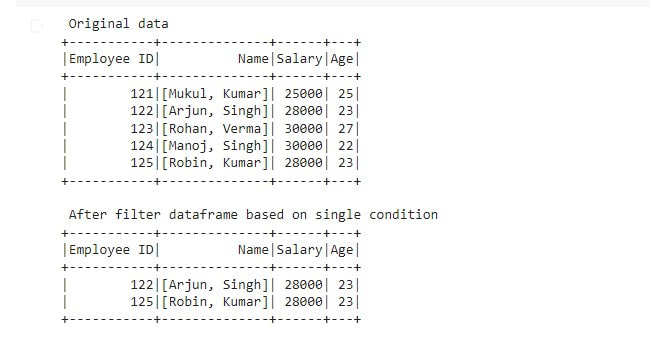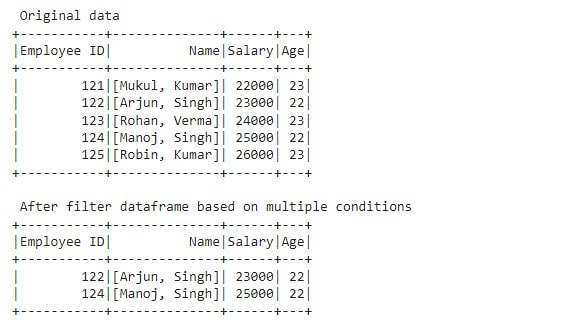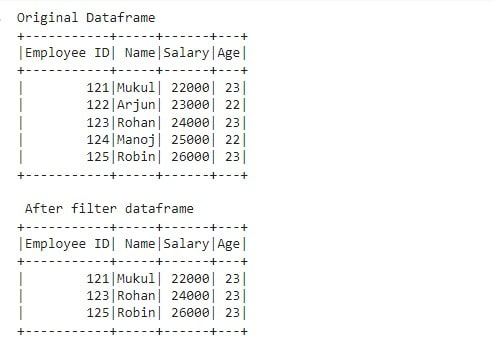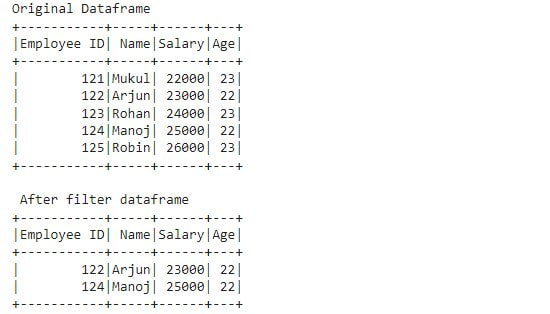# PySpark DataFrame – Where Filter

• Last Updated : 28 Mar, 2022

In this article, we are going to see where filter in PySpark Dataframe. Where() is a method used to filter the rows from DataFrame based on the given condition. The where() method is an alias for the filter() method. Both these methods operate exactly the same. We can also apply single and multiple conditions on DataFrame columns using the where() method.

Syntax: DataFrame.where(condition)

Example 1:

The following example is to see how to apply a single condition on Dataframe using the where() method.

## Python3

 `# importing required module``import` `pyspark``from` `pyspark.sql ``import` `SparkSession``from` `pyspark.sql ``import` `functions as F`` ` `# creating sparksession and giving an app name``spark ``=` `SparkSession.builder.appName(``'sparkdf'``).getOrCreate()`` ` `# list of Employees data``data ``=` `[``    ``(``121``, (``"Mukul"``, ``"Kumar"``), ``25000``, ``25``),``    ``(``122``, (``"Arjun"``, ``"Singh"``), ``28000``, ``23``),``    ``(``123``, (``"Rohan"``, ``"Verma"``), ``30000``, ``27``),``    ``(``124``, (``"Manoj"``, ``"Singh"``), ``30000``, ``22``),``    ``(``125``, (``"Robin"``, ``"Kumar"``), ``28000``, ``23``)``]`` ` `# specify column names``columns ``=` `[``'Employee ID'``, ``'Name'``, ``'Salary'``, ``'Age'``]`` ` `# creating a dataframe from the lists of data``df ``=` `spark.createDataFrame(data, columns)``print``(``" Original data "``)``df.show()`` ` `# filter dataframe based on single condition``df2 ``=` `df.where(df.Salary ``=``=` `28000``)``print``(``" After filter dataframe based on single condition  "``)``df2.show()`

Output:Example 2:

The following example is to understand how to apply multiple conditions on Dataframe using the where() method.

## Python3

 `# importing required module``import` `pyspark``from` `pyspark.sql ``import` `SparkSession``from` `pyspark.sql ``import` `functions as F`` ` `# creating sparksession and giving an app name``spark ``=` `SparkSession.builder.appName(``'sparkdf'``).getOrCreate()`` ` `# list of Employees data``data ``=` `[``    ``(``121``, (``"Mukul"``, ``"Kumar"``), ``22000``, ``23``),``    ``(``122``, (``"Arjun"``, ``"Singh"``), ``23000``, ``22``),``    ``(``123``, (``"Rohan"``, ``"Verma"``), ``24000``, ``23``),``    ``(``124``, (``"Manoj"``, ``"Singh"``), ``25000``, ``22``),``    ``(``125``, (``"Robin"``, ``"Kumar"``), ``26000``, ``23``)``]`` ` `# specify column names``columns ``=` `[``'Employee ID'``, ``'Name'``, ``'Salary'``, ``'Age'``]`` ` `# creating a dataframe from the lists of data``df ``=` `spark.createDataFrame(data, columns)``print``(``" Original data "``)``df.show()`` ` `# filter dataframe based on multiple conditions``df2 ``=` `df.where((df.Salary > ``22000``) & (df.Age ``=``=` `22``))``print``(``" After filter dataframe based on multiple conditions  "``)``df2.show()`

Output:Example 3:

The following example is to know how to filter Dataframe using the where() method with Column condition. We will use where() methods with specific conditions.

## Python3

 `# importing required module``import` `pyspark``from` `pyspark.sql ``import` `SparkSession``from` `pyspark.sql ``import` `functions as F`` ` `# creating sparksession and giving an app name``spark ``=` `SparkSession.builder.appName(``'sparkdf'``).getOrCreate()`` ` `# list of Employees data``data ``=` `[``    ``(``121``, ``"Mukul"``, ``22000``, ``23``),``    ``(``122``, ``"Arjun"``, ``23000``, ``22``),``    ``(``123``, ``"Rohan"``, ``24000``, ``23``),``    ``(``124``, ``"Manoj"``, ``25000``, ``22``),``    ``(``125``, ``"Robin"``, ``26000``, ``23``)``]`` ` `# specify column names``columns ``=` `[``'Employee ID'``, ``'Name'``, ``'Salary'``, ``'Age'``]`` ` `# creating a dataframe from the lists of data``df ``=` `spark.createDataFrame(data, columns)``print``(``"Original Dataframe"``)``df.show()`` ` `# where() method with SQL Expression``df2 ``=` `df.where(df[``"Age"``] ``=``=` `23``)``print``(``" After filter dataframe"``)``df2.show()`

Output:Example 4:

The following example is to know how to use where() method with SQL Expression.

## Python3

 `# importing required module``import` `pyspark``from` `pyspark.sql ``import` `SparkSession``from` `pyspark.sql ``import` `functions as F`` ` `# creating sparksession and giving an app name``spark ``=` `SparkSession.builder.appName(``'sparkdf'``).getOrCreate()`` ` `# list of Employees data``data ``=` `[``    ``(``121``, ``"Mukul"``, ``22000``, ``23``),``    ``(``122``, ``"Arjun"``, ``23000``, ``22``),``    ``(``123``, ``"Rohan"``, ``24000``, ``23``),``    ``(``124``, ``"Manoj"``, ``25000``, ``22``),``    ``(``125``, ``"Robin"``, ``26000``, ``23``)``]`` ` `# specify column names``columns ``=` `[``'Employee ID'``, ``'Name'``, ``'Salary'``, ``'Age'``]`` ` `# creating a dataframe from the lists of data``df ``=` `spark.createDataFrame(data, columns)``print``(``"Original Dataframe"``)``df.show()`` ` `# where() method with SQL Expression``df2 ``=` `df.where(``"Age == 22"``)``print``(``" After filter dataframe"``)``df2.show()`

Output:My Personal Notes arrow_drop_up